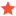(Python API) Mat.Offset() pose formatj_csoMemberPosts: 32 Threads: 13 Joined: May 2022 Reputation: 0 09-29-2022, 02:53 AM 1. Documentation bug in https://robodk.com/doc/en/PythonAPI/robo...Mat.Offset : "Calculates a relative target with respect to the reference frame coordinates. X,Y,Z are in mm, W,P,R are in degrees." Arguments are rx, ry, rz, not W, P, R.  Not to be confused with https://robodk.com/doc/en/PythonAPI/robo...ath.Offset which correctly lists rx,ry,rz. 2. As far as I can tell, Offset() uses a pose format unsupported by all the Pose_2_* functions, in that it wants rx,ry,rz in degrees.  The closest supported pose I found is Pose_2_TxyzRxyz(), which returns rx,ry,rz in radians.  So if I want to offset 2 poses relative to each other, I have to convert radians to degrees: Code:```    a = robodk.Pose(100, 200, 300, 30, 45, 60)     b = robodk.Pose(10, 0, 0, 0, 0, 0)     x, y, z, rx_radians, ry_radians, rz_radians = robomath.Pose_2_TxyzRxyz(b)     rx_degrees = math.degrees(rx_radians)     ry_degrees = math.degrees(ry_radians)     rz_degrees = math.degrees(rz_radians)     c = a.Offset(x, y, z, rx_degrees, ry_degrees, rz_degrees)``` It's also weird that Offset() wants broken out x,y,z,rx,ry,rz instead of taking a Pose: a.Offset(b). 3. If I have a reference frame with Pose f and its child target with Pose t, what's the equivalent math?  After a bunch of messing around with Offset(), looks like it's just f * t.  I can also get the same answer with the code above, but since most of the people looking at Offset() are probably trying to do what I was with two Poses, it's probably worth mentioning that they just need to multiply the poses together. Find ReplySamModeratorPosts: 181 Threads: 1 Joined: Jun 2021 Reputation: 7 09-29-2022, 11:59 AM 1. I have updated the documentation, it should reflect the change in the next publication. 2 and 3. If the two poses are known, we typically use new_pose = pose_a * pose_b directly. Offset() is convenient on loops, for instance: Code:```for i in range (10):  pose_i = pose.Offset(0, 0, 0, rz=i*5)``` Note that Pose_2_Staubli returns Pose_2_TxyzRxyz in degrees. Find useful information about RoboDK and its features by visiting our Online Documentation and by watching tutorials on our YouTube Channel. Find Reply